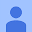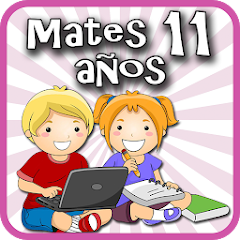# Matemáticas 11 años

3.4
845 reviews
100K+Everyone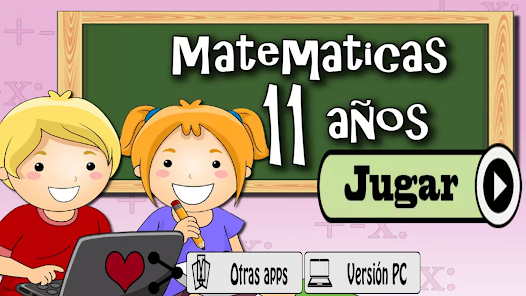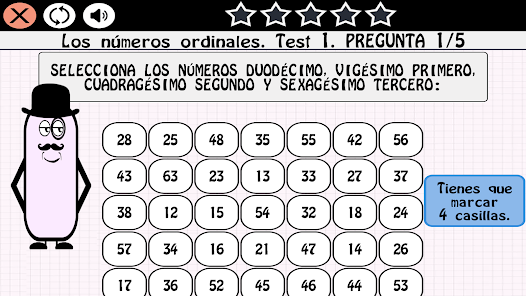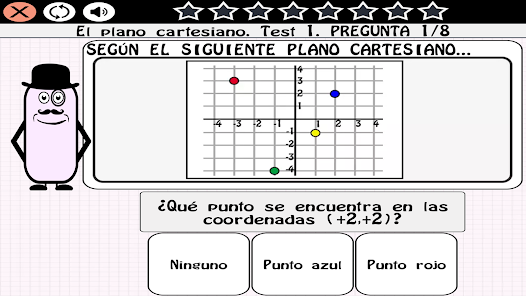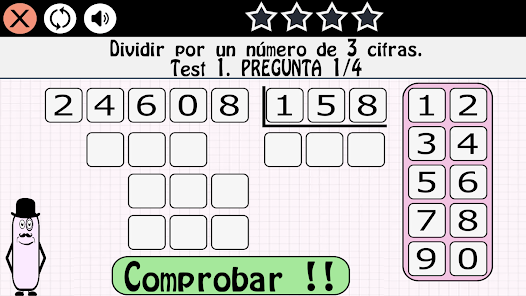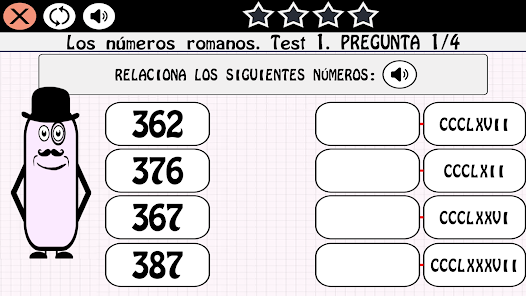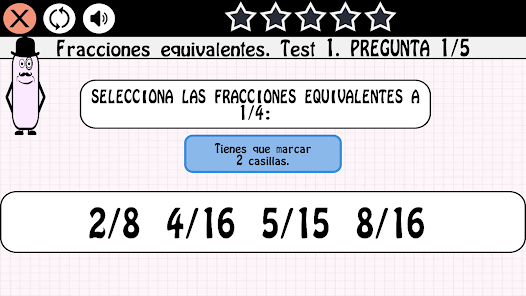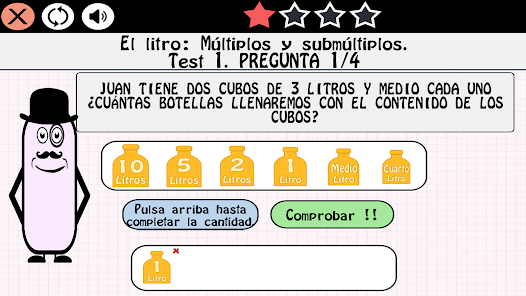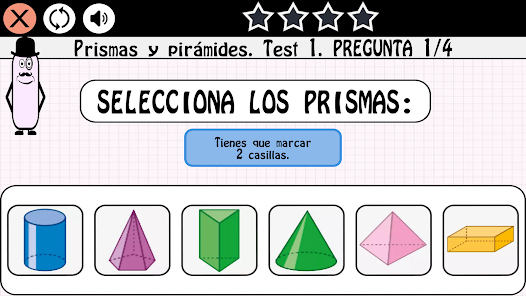Review the MATH syllabus for 11-year-old children with our fun application, completing the subject map until you take the final exam.

- Totally free.
- Specially designed for children in sixth grade.
- More than 1000 questions.
- Final exam.
- Easy to play.
- Possibility to play without Internet.

Syllabus:
Natural numbers and operations: 8-digit numbers, Roman numerals, Ordinal numbers, Approximation by rounding, Properties of addition and relationship with subtraction, Multiplication by two digits, Multiplication problems, Dividing a 4-digit number by another of 2, Divide by a 3-digit number and Combined Operations.
Powers and square root: Powers, squares and cubes, Powers of base 10, Polynomial decomposition of a number and Square root.
Divisibility: Multiples of a number, Least common multiple, Divisors of a number, Greatest common divisor, Prime and composite numbers.
Integers: Positive and negative numbers, Comparison and ordering of integers, The Cartesian plane, Addition of integers and Integer problems.
Decimal numbers and operations: Decimal numbers, Comparison and ordering of decimals, Addition and subtraction of decimal numbers, Multiplication of decimal numbers and Division of decimal numbers.
Fractions and operations: Fractions, The fraction of a quantity, Comparison of fractions, Equivalent fractions, Reduction to a common denominator, Addition and subtraction of fractions, Multiplication of fractions and Division of fractions.
Proportionality and percentages: Reduction to the unit, The rule of three, Percentages and Calculation of percentage of a quantity.
Sexagesimal system: Minutes and hours, Year, semesters, quarters and months, Years, decades, centuries and millennia, Complex and incomplex expressions of time measurements, Problems with amounts of time, Operations with amounts of time, The measure of angles and Operations with angle measures.
Metric system: Submultiples of the meter, Multiples of the meter, Complex and incomplex expressions of measures of length, Problems of measures of length, The liter: Multiples and submultiples, Complex and incomplex expressions of measures of capacity, The gram: Multiples and submultiples, Complex and incomplex expressions of mass measurements, Units of area and Units of volume.
Perimeter and area of ​​plane figures: Areas of the square, rectangle, rhombus and rhomboid, Perimeter and area of ​​the triangle, Length of the circumference and Area of ​​the circle.
Geometric bodies: Prisms and pyramids, Polyhedrons and Bodies of revolution.
Statistics and probability: Bar graphs, Mean, mode and range, Sure, possible, impossible and Probability of an event.

Enjoy our application at no cost and reinforce your knowledge of mathematics for children of 11 years.
Updated on
Jan 5, 2023

## Data safety

Safety starts with understanding how developers collect and share your data. Data privacy and security practices may vary based on your use, region, and age. The developer provided this information and may update it over time.No data shared with third partiesNo data collected